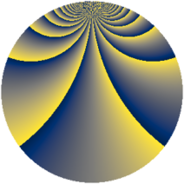# Properties

 Label 1045.2.cbLevel $1045$ Weight $2$ Character orbit 1045.cb Rep. character $\chi_{1045}(43,\cdot)$ Character field $\Q(\zeta_{36})$ Dimension $1392$ Sturm bound $240$

# Related objects

## Defining parameters

 Level: $$N$$ $$=$$ $$1045 = 5 \cdot 11 \cdot 19$$ Weight: $$k$$ $$=$$ $$2$$ Character orbit: $$[\chi]$$ $$=$$ 1045.cb (of order $$36$$ and degree $$12$$) Character conductor: $$\operatorname{cond}(\chi)$$ $$=$$ $$1045$$ Character field: $$\Q(\zeta_{36})$$ Sturm bound: $$240$$

## Dimensions

The following table gives the dimensions of various subspaces of $$M_{2}(1045, [\chi])$$.

Total New Old
Modular forms 1488 1488 0
Cusp forms 1392 1392 0
Eisenstein series 96 96 0

## Trace form

 $$1392 q - 24 q^{3} - 24 q^{5} + O(q^{10})$$ $$1392 q - 24 q^{3} - 24 q^{5} - 12 q^{11} - 12 q^{12} - 24 q^{15} - 48 q^{16} - 168 q^{20} - 24 q^{23} - 72 q^{25} + 96 q^{26} - 12 q^{27} - 24 q^{31} - 54 q^{33} - 48 q^{36} - 48 q^{37} + 144 q^{38} - 96 q^{42} - 12 q^{45} - 72 q^{47} - 144 q^{48} + 12 q^{53} - 78 q^{55} - 96 q^{56} + 96 q^{58} + 72 q^{60} - 72 q^{66} + 216 q^{67} - 120 q^{70} - 48 q^{71} - 48 q^{75} - 240 q^{77} - 108 q^{78} + 12 q^{80} - 144 q^{81} + 12 q^{82} - 48 q^{86} - 162 q^{88} + 96 q^{91} - 228 q^{92} + 276 q^{93} - 72 q^{97} + O(q^{100})$$

## Decomposition of $$S_{2}^{\mathrm{new}}(1045, [\chi])$$ into newform subspaces

The newforms in this space have not yet been added to the LMFDB.